Students can download Maths Chapter 5 Statistics Ex 5.2 Questions and Answers, Notes, Samacheer Kalvi 6th Maths Guide Pdf helps you to revise the complete Tamilnadu State Board New Syllabus, helps students complete homework assignments and to score high marks in board exams.

Tamilnadu Samacheer Kalvi 6th Maths Solutions Term 1 Chapter 5 Statistics Ex 5.2

Question 1.
Fill in the blanks.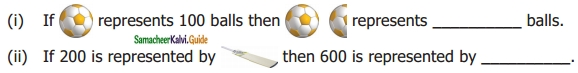(iii) Representation of data by using pictures is known as ………
Solution:
(i) 150
(ii)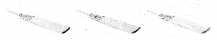(iii) PictographQuestion 2.
Draw a pictograph for the given data.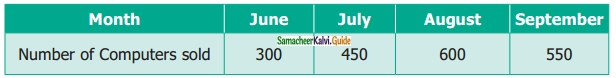Solution:
Number of computers sold
Scale : 1 Unit = 100 computers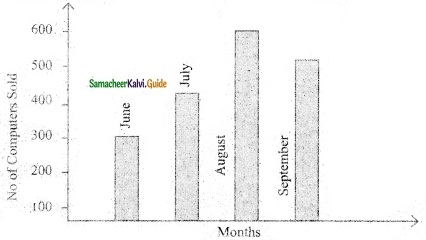Question 3.
The following table shows the number of tourists who visited the places in the month of May. Draw a pictograph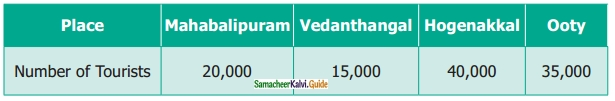Solution:
Number of Tourists who visited the places in the month of May.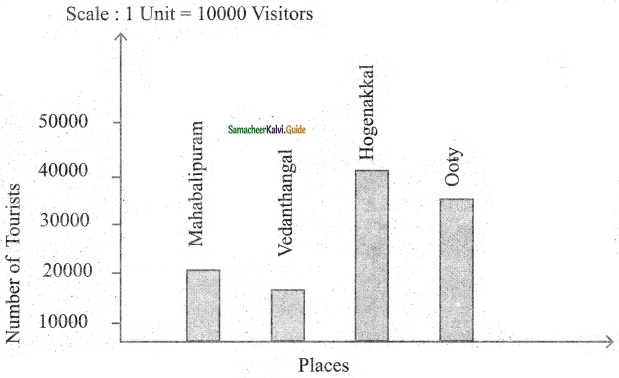Question 4.
The following pictograph shows the number of students playing different games in a school.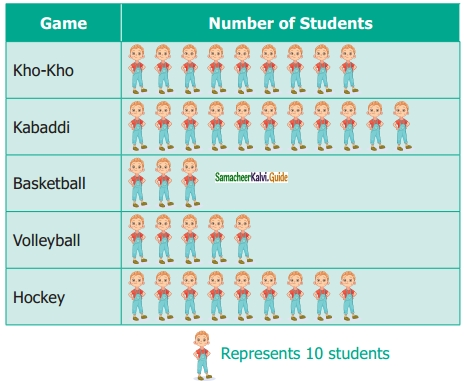(i) Which is the most popular game among the students?
(ii) Find the number of students playing Kabaddi.
(iii) Which two games are played by an equal number of students?
(iv) What is the difference between the number of students playing Kho-Kho and Hockey?
(v) Which is the least popular game among the students?
Solution:
(i) Kabaddi is the most popular game among students.
(ii) There are 11 × 10 = 110 students playing kabaddi.
(iii) Kho-Kho and Hockey are played by an equal number of students.
(iv) Difference is 90 – 90 = 0.
(v) Basketball is the least popular game among students.Objective Type Questions

Question 5.
The pictorial representation for a phrase is a ____
(a) Picto
(b) Tally mark
(c) Frequency
(d) Data
Solution:
(d) Data

Question 6.
A pictograph is also known as
a) Pictoword
(b) Pictogram
(c) Pictophrase
(d) Pictograph
Solution:
(b) Pictogram